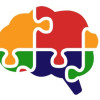## Available courses

### Foundations of Python Programming•Hetedu System

### Foundations of Python Programming

Develop the skills and logical thinking to create programs and projects in Python, using strings, lists, loops, dictionaries and functions.### Foundations of the NumPy Package•Hetedu System

### Foundations of the NumPy Package

Develop the skills to carry out the analysis of data sets using linear algebra and complex mathematical operations with the NumPy package.### Foundations of the Pandas Dataframe Package•Hetedu System

### Foundations of the Pandas Dataframe Package

Develop the skills to obtain, transform, load and analyze data sets using the Pandas package, which makes it easy to manage all types of data in two-dimensional arrays and tables with access to rows and columns.### Database Programming Fundamentals with SQL•Hetedu System

### Database Programming Fundamentals with SQL

Develop the technical skills required to implement and manage database solutions with Microsoft SQL Server.### NoSQL Database Programming Fundamentals•Hetedu System

### NoSQL Database Programming Fundamentals

Develop the technical skills required to implement and manage NoSQL database solutions.### Data Wrangling•Hetedu System

### Data Wrangling

Improve skills in performing data analysis, manipulation and ETL processes with the Pandas package.### Data Visualization with Python•Hetedu System

### Data Visualization with Python

Develop the skills to create visuals that show the data history, trends, dashboard, and other visual resources with the matplotlib and seaborn packages.### Statistics and Probability•Hetedu System

### Statistics and Probability

Learn the different probabilistic models and apply statistical inference in real scenarios, building supervised learning models.### Data Visualization and Analysis with Power BI•Hetedu System

### Data Visualization and Analysis with Power BI

Provide practical experience to be applied in data analysis with Power BI, acquiring and developing the skills required to identify business requirements, identify and acquire data. Also transform data, create data models, visualization objects, and share resources using Power BI services.### Foundations of Python Programming•Hetedu System

### Foundations of Python Programming

Develop the skills and logical thinking to create programs and projects in Python, using strings, lists, loops, dictionaries and functions.### Foundations of the NumPy Package•Hetedu System

### Foundations of the NumPy Package

Develop the skills to carry out the analysis of data sets using linear algebra and complex mathematical operations with the NumPy package.### Foundations of the Dataframe Package•Hetedu System

### Foundations of the Panda Dataframe Package

Develop the skills to obtain, transform, load and analyze data sets using the Pandas package, which makes it easy to manage all types of data in two-dimensional arrays and tables with access to rows and columns.### Data Wrangling•Hetedu System

### Data Wrangling

Improve skills in performing data analysis, manipulation and ETL processes with the Pandas package.### Data Visualization with Python•Hetedu System

### Data Visualization with Python

Develop the skills to create visuals that show the data history, trends, dashboard, and other visual resources with the matplotlib and seaborn packages.### Statistics and Probability•Hetedu System

### Statistics and Probability

Learn the different probabilistic models and apply statistical inference in real scenarios, building supervised learning models.### Data Visualization and Analysis with Power BI•Hetedu System

### Data Visualization and Analysis with Power BI

Provide practical experience to be applied in data analysis with Power BI, acquiring and developing the skills required to identify business requirements, identify and acquire data. Also transform data, create data models, visualization objects, and share resources using Power BI services.### Differential Calculus and Numerical Differentiation•Hetedu System

### Differential Calculus and Numerical Differentiation

Understand and apply the mathematical concepts of differential calculus in the study of physical and engineering phenomena.### Integral Calculus and Numerical Integration•Hetedu System

### Integral Calculus and Numerical Integration

Develop mathematical concepts to carry out the integration analytically that would yield exact solutions and using numerical methods that would yield approximate solutions, considering the approximation error.### Multivariable Calculus•Hetedu System

### Multivariable Calculus

Apply the mathematical concepts of multivariable calculus in the study of physical and engineering phenomena, to model and study high-dimensional systems that exhibit deterministic behavior.### Introduction to Linear Algebra•Hetedu System

### Introduction to Linear Algebra

The course is aimed at the study of linear and nonlinear systems to solve engineering and applied science problems, through the modeling of natural phenomena and models of industrial machines, improving the ability to efficiently compute these models.### Interpolation and Regression Methods•Hetedu System

### Interpolation and Regression Methods

Apply interpolation and regression models to make estimates and build predictive models based on historical data and mathematical functions.### Differential Equations with Numerical Solutions•Hetedu System

### Differential Equations with Numerical Solutions

Understand and apply the mathematical concepts of differential equations and the Laplace transform to solve problems in applied sciences and engineering, as well as develop programs and simulators with Python.